# Builtins Module - Built-in Model Classes¶

This module contains builtin function models that use the framework of the core module.

## Class Inheritance Diagram¶

builtins includes a number of classes that are subclasses of classes from the core module. The relationship between these classes is shown in the diagram below.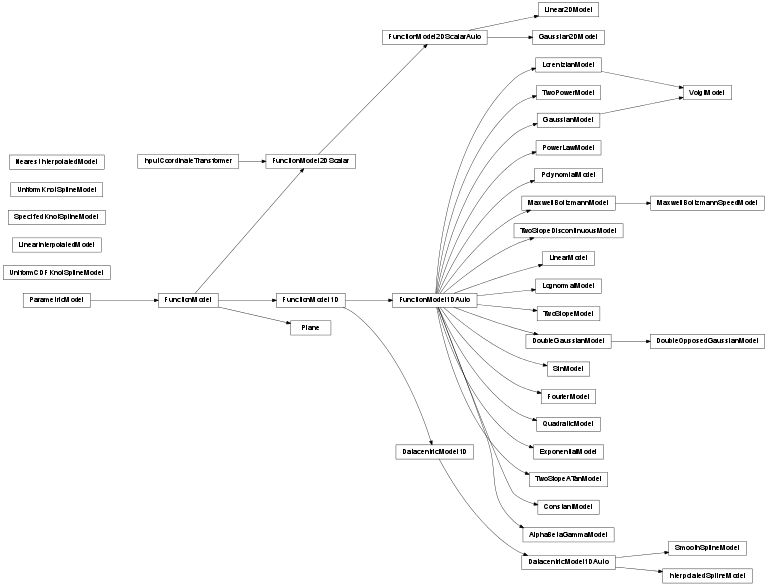## Module Reference¶

### Classes¶

 ConstantModel The simplest model imaginable - just a constant value. LinearModel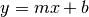linear model. QuadraticModel 2-degree polynomial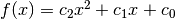PolynomialModel Arbitrary-degree polynomial FourierModel A Fourier model composed of sines and cosines: GaussianModel Normalized 1D gaussian function: DoubleGaussianModel Sum of two normalized 1D gaussian functions. Note that fitting often can be DoubleOpposedGaussianModel This model is a DoubleGaussianModel that forces one of the gaussians to LognormalModel A normalized Lognormal model LorentzianModel A standard Lorentzian function (also known as the Cauchy distribution): VoigtModel A Voigt model constructed as the convolution of a GaussianModel and ExponentialModel exponential function Ae^(kx) PowerLawModel A single power law model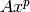SinModel A trigonometric model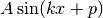TwoPowerModel A model that smoothly transitions between two power laws. TwoSlopeModel This model smoothly transitions from linear with one slope to linear with a different slope. TwoSlopeDiscontinuousModel This model discontinuously transitions between two slopes and is linear everywhere else. TwoSlopeATanModel This model transitions between two asymptotic slopes with an additional parameter that allows for a variable transition region size. LinearInterpolatedModel(**kwargs) A model that is the linear interpolation of the data, or if out of bounds, fixed to the edge value. NearestInterpolatedModel(**kwargs) A model that is the interpolation of the data by taking the value of the SmoothSplineModel(**kwargs) This model uses a B-spline as a model for the function. Note that by default InterpolatedSplineModel() This uses a B-spline as a model for the function. Note that by default the UniformKnotSplineModel() A spline model with a uniform seperation between the internal knots, with UniformCDFKnotSplineModel() A spline model with a seperation between the internal knots set uniformly on SpecifiedKnotSplineModel() AlphaBetaGammaModel This model is a generic power-law-like model of the form .. MaxwellBoltzmannModel A Maxwell-Boltzmann distribution for 1D velocity: MaxwellBoltzmannSpeedModel A Maxwell-Boltzmann distribution for 3D average speed: Gaussian2DModel Two dimensional Gaussian model (not normalized - peak value is 1). Linear2DModel A simple model that is simply the linear combination of the two inputs. Plane([varorder, vn, wn, origin]) Models a plane of the form ..